### We take levels for granted.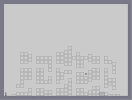Hover over the thumbnail for a full-size version.

Author NachoCheese author:nachocheese nreality rated 2011-05-06 2011-05-06 4 by 9 people. \$We take levels for granted.#NachoCheese#nreality#00000000000000000000000000000000000000000000000000000000000000000000000000000000000000000000000000000000000000000000000000000000000000000000000000000000000000000000000000000000000000000000000000000000000000000000000000000000000000000000000000000000000000000000000000000000000000000000000000000000000000000000000000000000000000000000000000000000000000000000000000000000000000000000000000000000000000000000000000000000000000000000000000000000000000000000000000000000000000000000000000000000000000000000000000000000000000000000000000000000000000000000000000000000000000000000000000000000000000000000000000000000000000000000000000000000000000000000000000000000000000000000000000000000000000000000000000000000000000000|5^36,564!1^84,576^^^84.588,8,9,0.25!1^108,564^^^108.588,8,9,0.25!1^108,516^^^108.588,8,9,0.25!1^132,564^^^132.588,8,9,0.25!1^132,516^^^132.588,8,9,0.25!1^132,492^^^132.588,8,9,0.25!1^132,468^^^132.588,8,9,0.25!1^132,444^^^132.588,8,9,0.25!1^132,420^^^132.588,8,9,0.25!1^132,372^^^132.588,8,9,0.25!1^132,348^^^132.588,8,9,0.25!1^132,324^^^132.588,8,9,0.25!1^156,564^^^156.588,8,9,0.25!1^156,468^^^156.588,8,9,0.25!1^156,444^^^156.588,8,9,0.25!1^156,420^^^156.588,8,9,0.25!1^156,372^^^156.588,8,9,0.25!1^156,348^^^156.588,8,9,0.25!1^156,324^^^156.588,8,9,0.25!1^180,564^^^180.588,8,9,0.25!1^180,540^^^180.588,8,9,0.25!1^180,468^^^180.588,8,9,0.25!1^180,444^^^180.588,8,9,0.25!1^180,420^^^180.588,8,9,0.25!1^180,360^^^180.588,8,9,0.25!1^180,336^^^180.588,8,9,0.25!1^228,564^^^228.588,8,9,0.25!1^228,540^^^228.588,8,9,0.25!1^228,492^^^228.588,8,9,0.25!1^228,468^^^228.588,8,9,0.25!1^228,444^^^228.588,8,9,0.25!1^228,420^^^228.588,8,9,0.25!1^228,372^^^228.588,8,9,0.25!1^228,348^^^228.588,8,9,0.25!1^252,564^^^252.588,8,9,0.25!1^252,540^^^252.588,8,9,0.25!1^252,492^^^252.588,8,9,0.25!1^252,468^^^252.588,8,9,0.25!1^252,444^^^252.588,8,9,0.25!1^252,420^^^252.588,8,9,0.25!1^252,372^^^252.588,8,9,0.25!1^252,348^^^252.588,8,9,0.25!1^276,576^^^276.588,8,9,0.25!1^276,552^^^276.588,8,9,0.25!1^276,492^^^276.588,8,9,0.25!1^276,372^^^276.588,8,9,0.25!1^276,348^^^276.588,8,9,0.25!1^300,576^^^300.588,8,9,0.25!1^300,552^^^300.588,8,9,0.25!1^300,492^^^300.588,8,9,0.25!1^300,468^^^300.588,8,9,0.25!1^300,420^^^300.588,8,9,0.25!1^300,396^^^300.588,8,9,0.25!1^300,372^^^300.588,8,9,0.25!1^300,348^^^300.588,8,9,0.25!1^348,564^^^348.588,8,9,0.25!1^348,540^^^348.588,8,9,0.25!1^348,492^^^348.588,8,9,0.25!1^348,468^^^348.588,8,9,0.25!1^348,444^^^348.588,8,9,0.25!1^348,420^^^348.588,8,9,0.25!1^348,372^^^348.588,8,9,0.25!1^348,348^^^348.588,8,9,0.25!1^348,324^^^348.588,8,9,0.25!1^372,564^^^372.588,8,9,0.25!1^372,540^^^372.588,8,9,0.25!1^372,468^^^372.588,8,9,0.25!1^372,444^^^372.588,8,9,0.25!11^612,564,516,444^^^516.588,8,9,0.25!1^372,372^^^372.588,8,9,0.25!1^372,348^^^372.588,8,9,0.25!1^372,324^^^372.588,8,9,0.25!1^396,564^^^396.588,8,9,0.25!1^396,540^^^396.588,8,9,0.25!1^396,516^^^396.588,8,9,0.25!1^396,432^^^396.588,8,9,0.25!1^396,384^^^396.588,8,9,0.25!1^396,360^^^396.588,8,9,0.25!1^396,336^^^396.588,8,9,0.25!1^396,312^^^396.588,8,9,0.25!1^420,576^^^420.588,8,9,0.25!1^420,552^^^420.588,8,9,0.25!1^420,528^^^420.588,8,9,0.25!1^420,504^^^420.588,8,9,0.25!1^420,480^^^420.588,8,9,0.25!1^420,432^^^420.588,8,9,0.25!1^420,384^^^420.588,8,9,0.25!1^420,360^^^420.588,8,9,0.25!1^420,336^^^420.588,8,9,0.25!1^420,312^^^420.588,8,9,0.25!1^420,288^^^420.588,8,9,0.25!1^468,564^^^468.588,8,9,0.25!1^468,540^^^468.588,8,9,0.25!1^468,492^^^468.588,8,9,0.25!1^468,468^^^468.588,8,9,0.25!1^468,444^^^468.588,8,9,0.25!1^468,396^^^468.588,8,9,0.25!1^468,372^^^468.588,8,9,0.25!1^468,348^^^468.588,8,9,0.25!1^468,324^^^468.588,8,9,0.25!1^492,564^^^492.588,8,9,0.25!1^492,540^^^492.588,8,9,0.25!1^492,492^^^492.588,8,9,0.25!1^492,468^^^492.588,8,9,0.25!1^492,444^^^492.588,8,9,0.25!1^492,396^^^492.588,8,9,0.25!1^492,372^^^492.588,8,9,0.25!1^492,348^^^492.588,8,9,0.25!1^516,564^^^516.588,8,9,0.25!1^516,492^^^516.588,8,9,0.25!1^516,468^^^516.588,8,9,0.25!1^540,576^^^540.588,8,9,0.25!1^540,552^^^540.588,8,9,0.25!1^540,480^^^540.588,8,9,0.25!1^540,456^^^540.588,8,9,0.25!1^540,432^^^540.588,8,9,0.25!1^540,360^^^540.588,8,9,0.25!1^540,336^^^540.588,8,9,0.25!1^564,576^^^564.588,8,9,0.25!1^564,552^^^564.588,8,9,0.25!1^564,528^^^564.588,8,9,0.25!1^564,468^^^564.588,8,9,0.25!1^564,444^^^564.588,8,9,0.25!1^564,420^^^564.588,8,9,0.25!1^564,372^^^564.588,8,9,0.25!1^564,348^^^564.588,8,9,0.25!1^588,576^^^588.588,8,9,0.25!1^588,552^^^588.588,8,9,0.25!1^588,528^^^588.588,8,9,0.25!1^588,468^^^588.588,8,9,0.25!1^588,444^^^588.588,8,9,0.25!1^588,420^^^588.588,8,9,0.25!1^588,372^^^588.588,8,9,0.25!1^588,348^^^588.588,8,9,0.25!1^636,564^^^636.588,8,9,0.25!1^636,516^^^636.588,8,9,0.25!1^636,492^^^636.588,8,9,0.25!1^636,468^^^636.588,8,9,0.25!1^636,444^^^636.588,8,9,0.25!1^636,420^^^636.588,8,9,0.25!1^636,372^^^636.588,8,9,0.25!1^636,348^^^636.588,8,9,0.25!1^660,576^^^660.588,8,9,0.25!1^660,504^^^660.588,8,9,0.25!1^660,480^^^660.588,8,9,0.25!1^660,372^^^660.588,8,9,0.25!1^684,576^^^684.588,8,9,0.25!1^684,528^^^684.588,8,9,0.25!1^684,504^^^684.588,8,9,0.25!1^684,480^^^684.588,8,9,0.25!1^684,432^^^684.588,8,9,0.25!1^684,408^^^684.588,8,9,0.25!1^684,384^^^684.588,8,9,0.25!1^708,576^^^708.588,8,9,0.25!1^516,348^^^516.588,8,9,0.25!1^444,348^^^444.588,8,9,0.25||||4,mass,1;4,stiff,1;# Yes, the shaking is intentional. And the slow-moving-ness. A teaser. Have fun everyone! :D

## Other maps by this author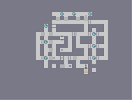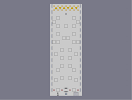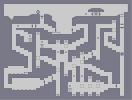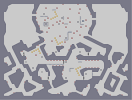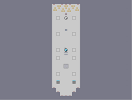The System. Taste the cake. Living in a lonely world The Labyrinth A feeling like floating. Dynamic Battlefield.

Pages: (0)

### Fastest

Hard to beat mystro, but I eventually was able to edge him out by a frame.
Demo Data 291:97587473|89480469|89478485|89478485|89478485|18175317|107374191|107374182|107374182|107374182|107374182|40265318|35791394|35791394|35791394|35791394|17826338|17895697|17895697|107335953|35792486|35791394|35791394|35791394|35791394|35791394|35791394|35791394|35791394|107374306|107374182|107374182|36071014|35791394|35791394|35791394|17825826|17895697|17895697|17|35651584|8738

### First Try

Didn't like the shaking, otherwise, I would love to see this expanded upon.
Demo Data 654:0|17895696|89478493|219223381|107234645|73819750|71582788|107373636|107374182|35792486|35791394|35791394|35791394|35791394|35791394|35791394|34|1118208|2236960|35791392|35791394|40296994|35791394|35791394|36071138|35791394|115483170|35791394|35791394|40267298|17895682|35782929|88501794|108483925|107376162|35791394|107880994|89474118|107374180|35792486|35791394|35791394|35791394|35791394|35791394|35791394|35791394|107880994|35808870|107374190|107374182|107374182|107880994|35808870|35791394|35791394|35791394|35791394|35791394|35791394|35791394|35791394|35791394|35791394|35791394|35791394|35791394|35791394|35791394|2236962|0|0|79691776|35791460|35791394|35791394|35791394|35791394|35791394|35791394|35791394|35791394|35791394|35791394|35791394|35791394|107376162|35791394|35791394|35791394|35791394|35791394|35791394|546
A bit laggy but great concept

### Can't play it.

My stupid slow computer is what I've had to resort too because my stupid fast computer stupid had a stupid hard drive problem that was stupid so my stupid old computer lags so stupidly stupid.
sub 300 completion, I love love love love this map....I even liked the shake, it made the map a little less predictable, and kept it from getting old. Also did I mention I loved this?
5Faved
Great fucking map!
Demo Data 292:17895680|97604957|89478485|89478485|89478485|89478485|105173269|107374182|107374182|107374182|40265318|35791394|35791394|35791394|35791394|35791394|35791394|16777250|17895697|69905|107374188|107374182|40265318|35791394|35791394|35791394|35791394|35791394|237117986|107374182|107374182|107374182|107374182|35792486|35791394|2236962|17895680|17895697|17895697|1|35791392|2
theNcreator speaks for me.

### I wouldn't say amazing

But certainly an improvement

### let me give you an idea

make this a tile and make non-chasing drones circulate around the city and make it sort of simple locksmith. that shit would be amazing

### I didnt like the shaking

but other than that, it was cool